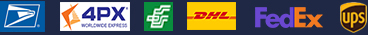•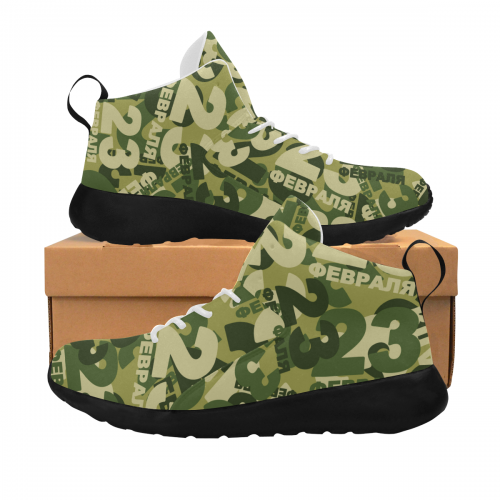•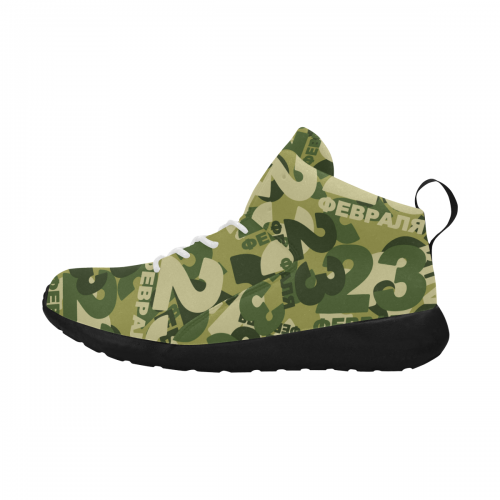•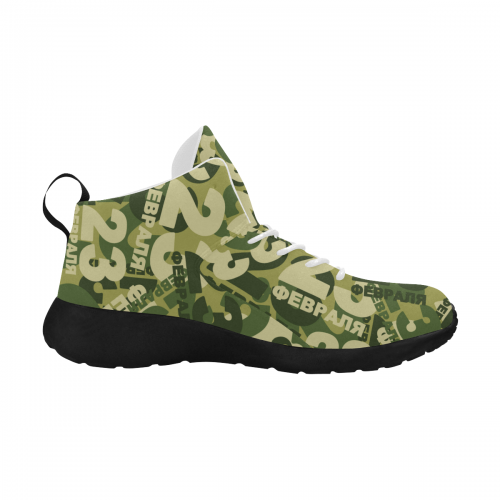•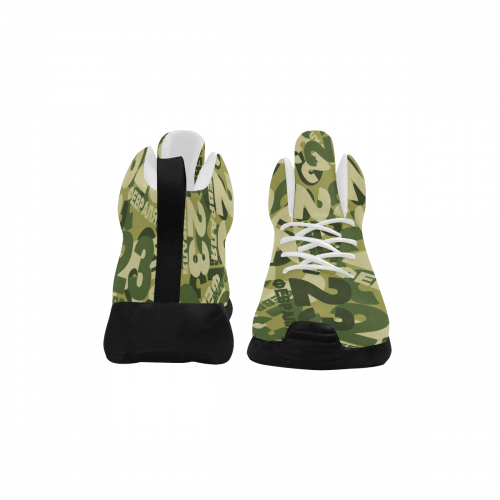### Men's Basketball Shoes (Model 57502)

\$37.99
Retail Price \$69.99

Partial Shipping Included

•   Black
•   White

EUR39 = US6 =UK5.5 = 253mm = 9.96inch

EUR40 = US7 =UK6= 259mm = 10.20inch
EUR41 = US8 =UK7 = 266mm =10.47inch
EUR42 = US8.5 =UK7.5 =273mm =10.74inch

EUR43 = US9.5 =UK8.5 =279mm = 10.98inch

EUR44 = US10=UK9=286mm =11.26inch
EUR45 = US11 =UK10 = 293mm =11.54inch
EUR46 = US12=UK11= 299mm =11.77inch

Payment methods we accpet: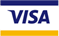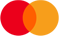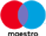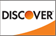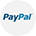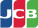We deliver with: